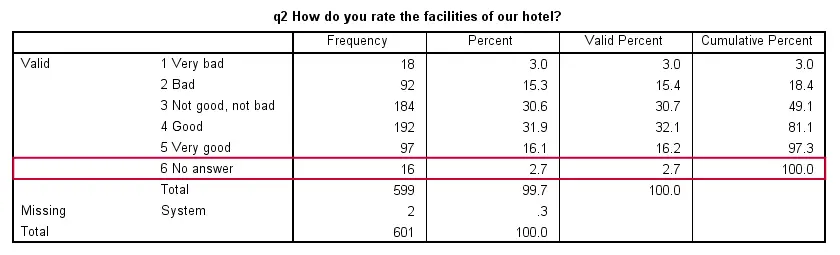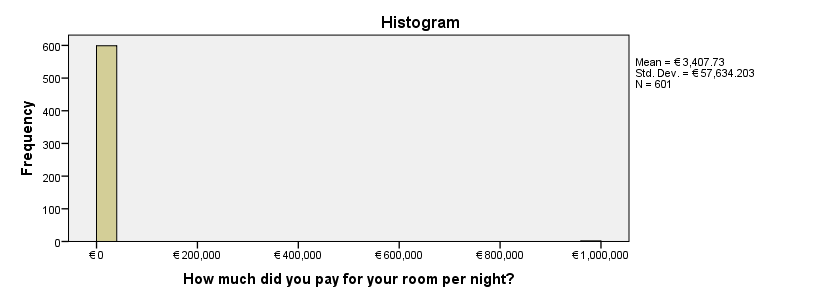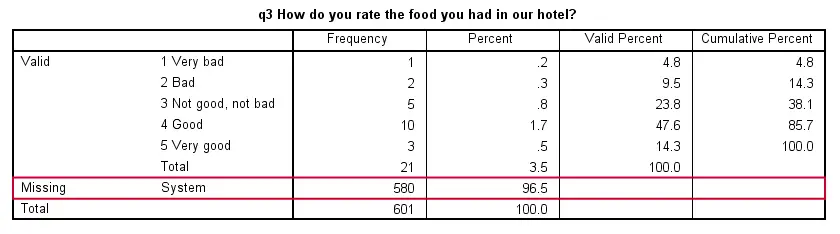SPSS TUTORIALS BASICS ANOVA REGRESSION FACTOR CORRELATION

# SPSS Data Preparation 4 – Specify Missing Values

## 4. Presence of User Missing Values

(Overview and data file are are found here)

User missing values are values that we want to exclude from analysis. We do so by specifying (ranges of) values as “missing” in SPSS. For ordinal variables, we typically exclude answers such as “Don't know” or “Not applicable”. For metric variables, we exclude values that are not plausible, usually extremely high or low values.
So how do we know whether a variable contains any values that we need to specify as missing? Well, for ordinal variables we run frequency tables with bar charts and for metric variable we run histograms. So let's see some examples.

## SPSS Frequency Table with Bar Chart Syntax

We first take a look at q2. Since this is an ordinal variable, we'll generate its frequency table and bar chart with the syntax below.

*1. Show values and value labels in tables.

set tnumbers both.

*2. Run frequency table and bar chart over q2.

frequencies q2/barchart.

## ResultFirst, note that higher values correspond to more positive attitudes regarding the hotel’s facilities. However, 6 (“No answer”) is not more positive than 5 (“Very good”). We therefore specify it as missing by running missing values q2 (6). If we now rerun our bar chart, we'll see that “No answer” is excluded from it as desired.

## SPSS Histogram Syntax

We'll now inspect whether we need to specify any user missings for rprice. Since it's a metric variable, we'll inspect its histogram by running frequencies rprice/histogram. The result, shown below, looks very weird; it seems as if some people paid €999,999 for their room. Also note that the average room price seems to be €3400 at this point.The problem here is that 999999 is probably a code indicating that the room price is unknown rather than €999,999. We'll therefore specify it as missing by running missing values rprice (999999). If we now rerun our histogram, it makes perfect sense and reports an average room price around €80.

## 5. Missing Values per Variable

(Overview and data file are are found here)

We previously proposed running frequency tables with bar charts for all categorical variables and histograms for all metric variables. We did so for checking whether any user missing values need to be specified. After doing so, we inspect the number of missing values (either user missing or system missing) for each variable. Variables having many missing values are often undesirable and are sometimes removed or excluded from analysis.
For example, let's inspect q3. Since it's an ordinal variable, we'll run a frequency table and bar chart with frequencies q3/barchart.

## ResultNote that 96.5% of all values are system missing. We have so few actual answers that we could consider dropping this variable altogether.

# Tell us what you think!

*Required field. Your comment will show up after approval from a moderator.